Courses

# The surfaces are frictionless, the ratio of T1 and T2 is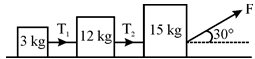a)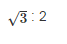b)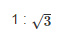c)1 : 5d)5 : 1Correct answer is option 'C'. Can you explain this answer? Related Test: NEET Mock Test - 1

## NEET Question

By Shubham Wagh · Feb 20, 2019 ·NEET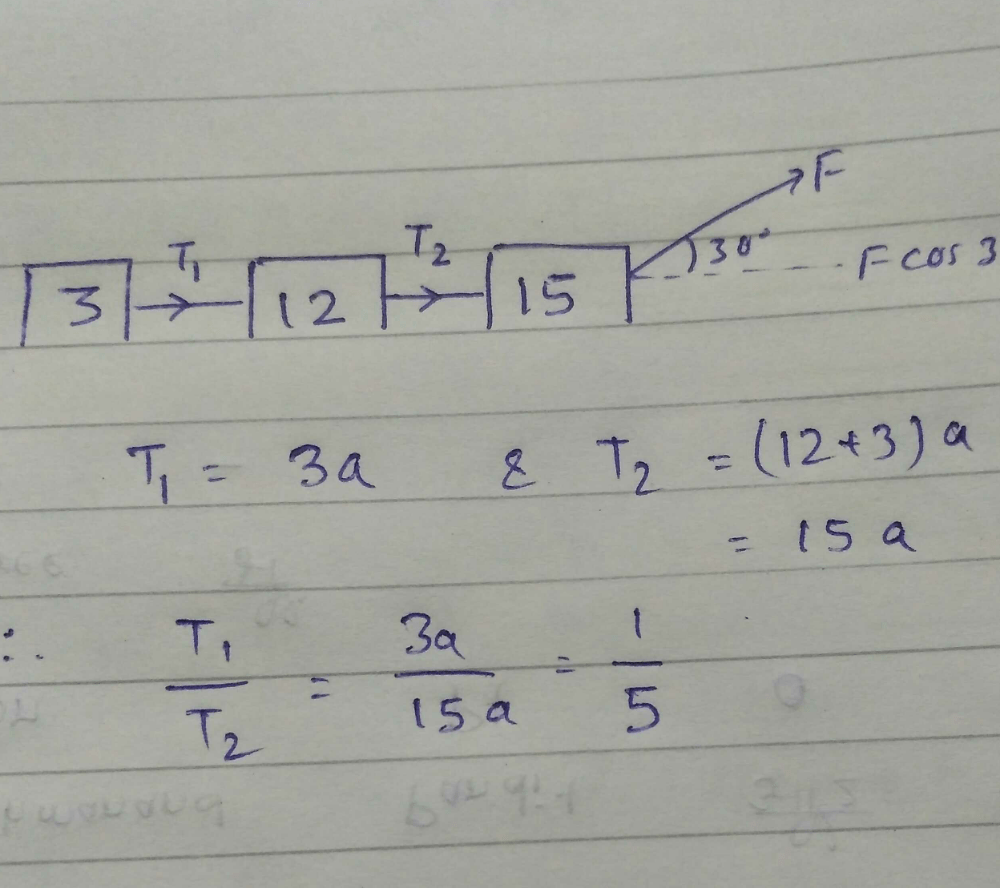Monika Rao answered Feb 21, 2019

Geeta Rayate answered Mar 30, 2020

Shristi Sharya answered Mar 30, 2020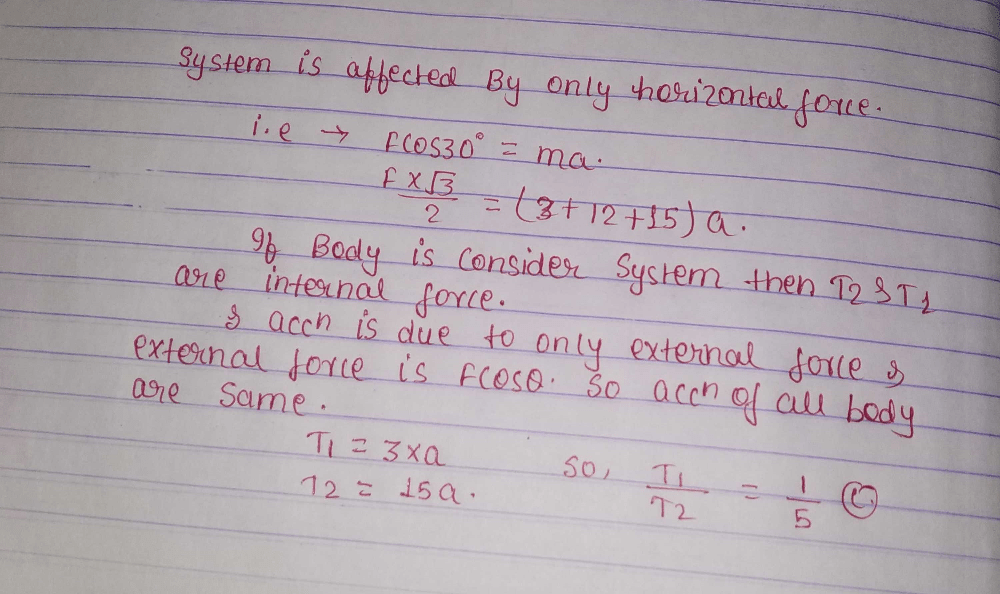Vijayam6309002257 Vijaya answered Apr 01, 2020
T1=3a,T2=(12+3)a
T1 /T2=3a/15a
=1/5

Anchal Maurya answered Feb 21, 2019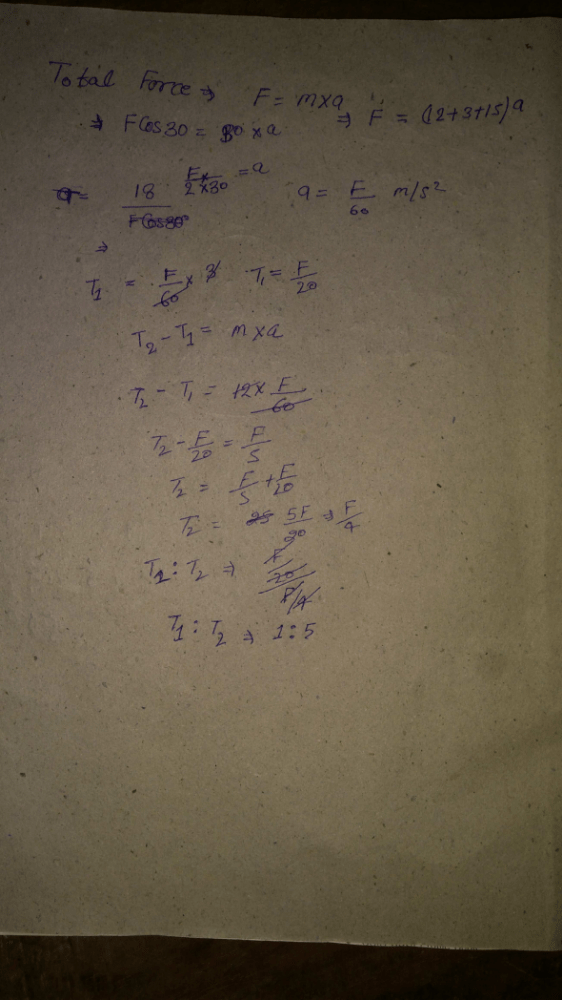Nirmala Devi answered Apr 01, 2020
T
1
= m1 a
T
2
= (m1 + m2)a
(T
1
/ T
2
) = {m1 / (m1 + m2)} = {3 / (3 + 12)} = (1 / 5)
hence T1 : T2 = 1:5